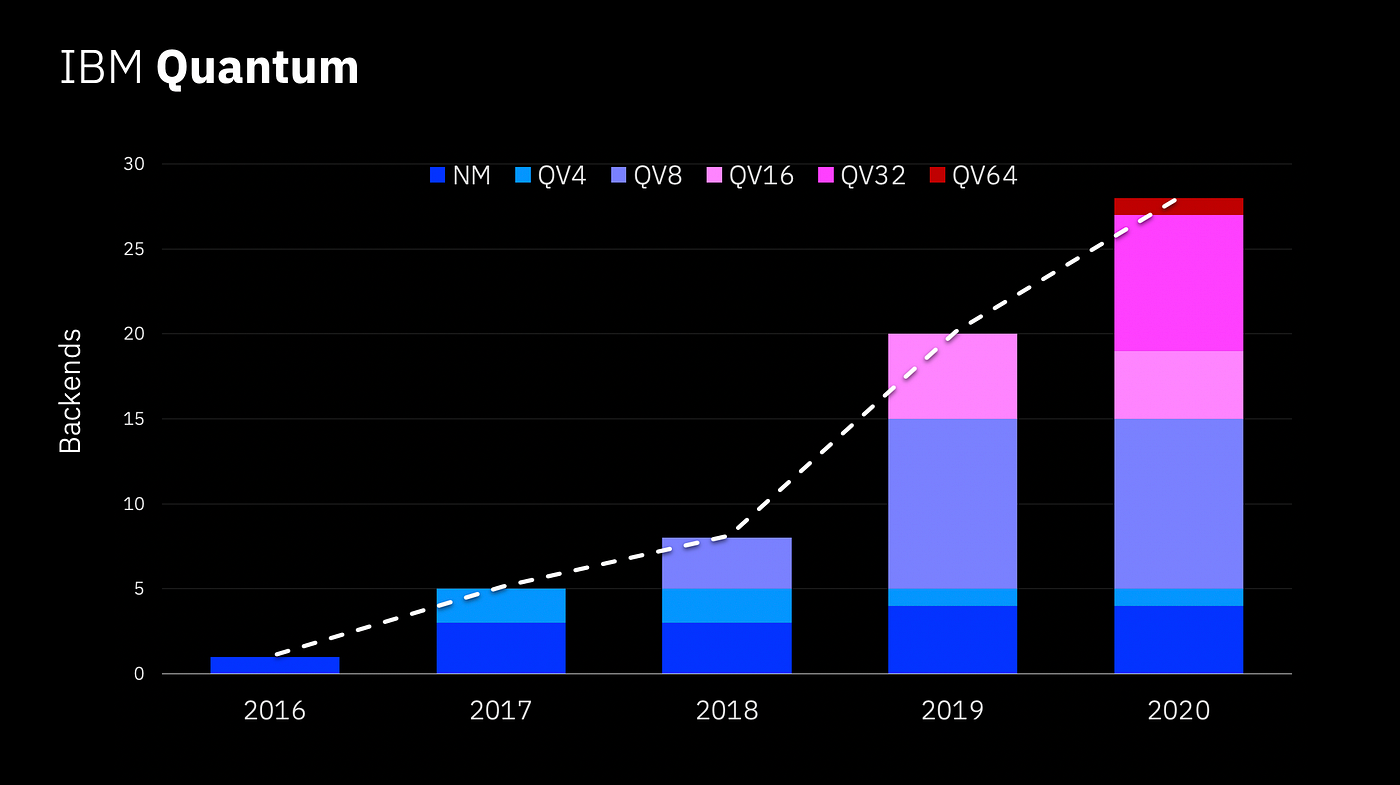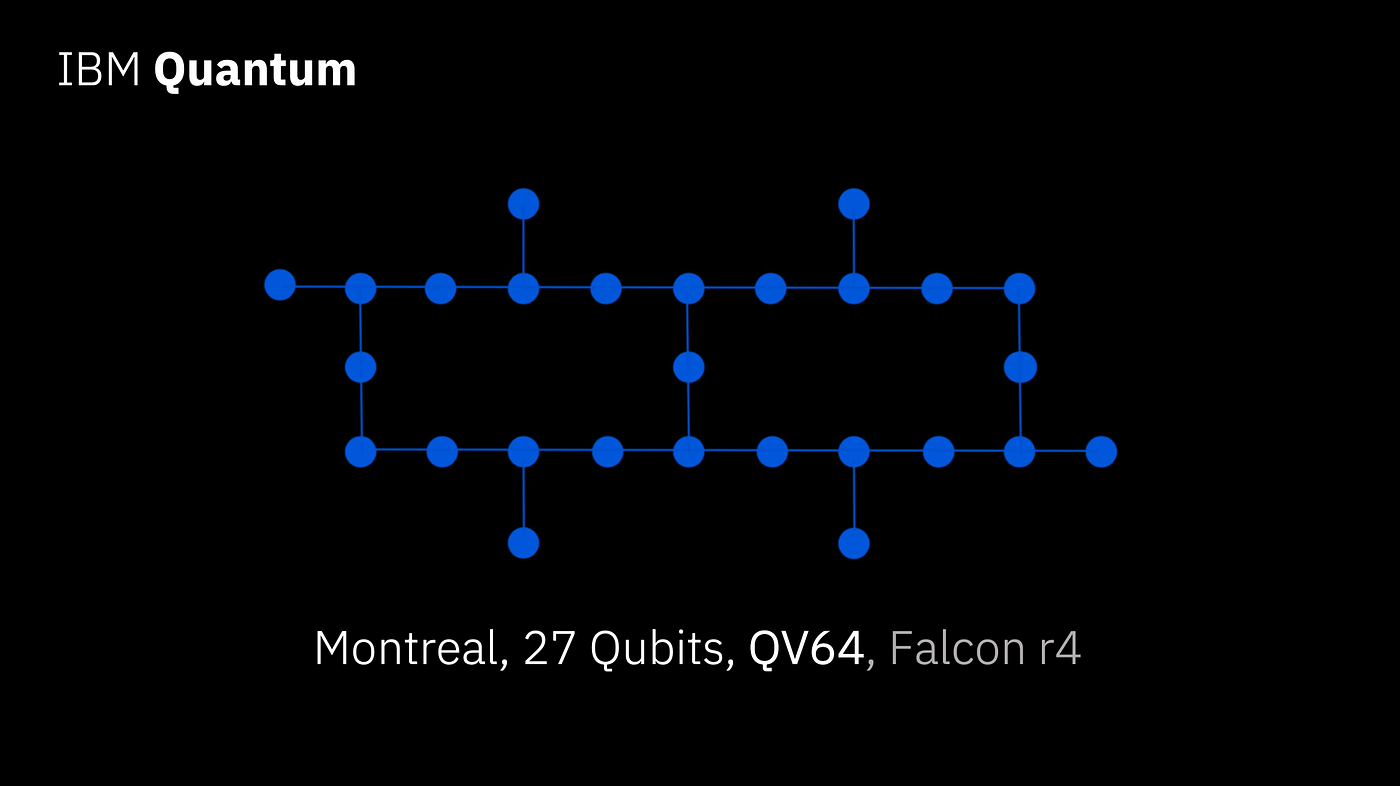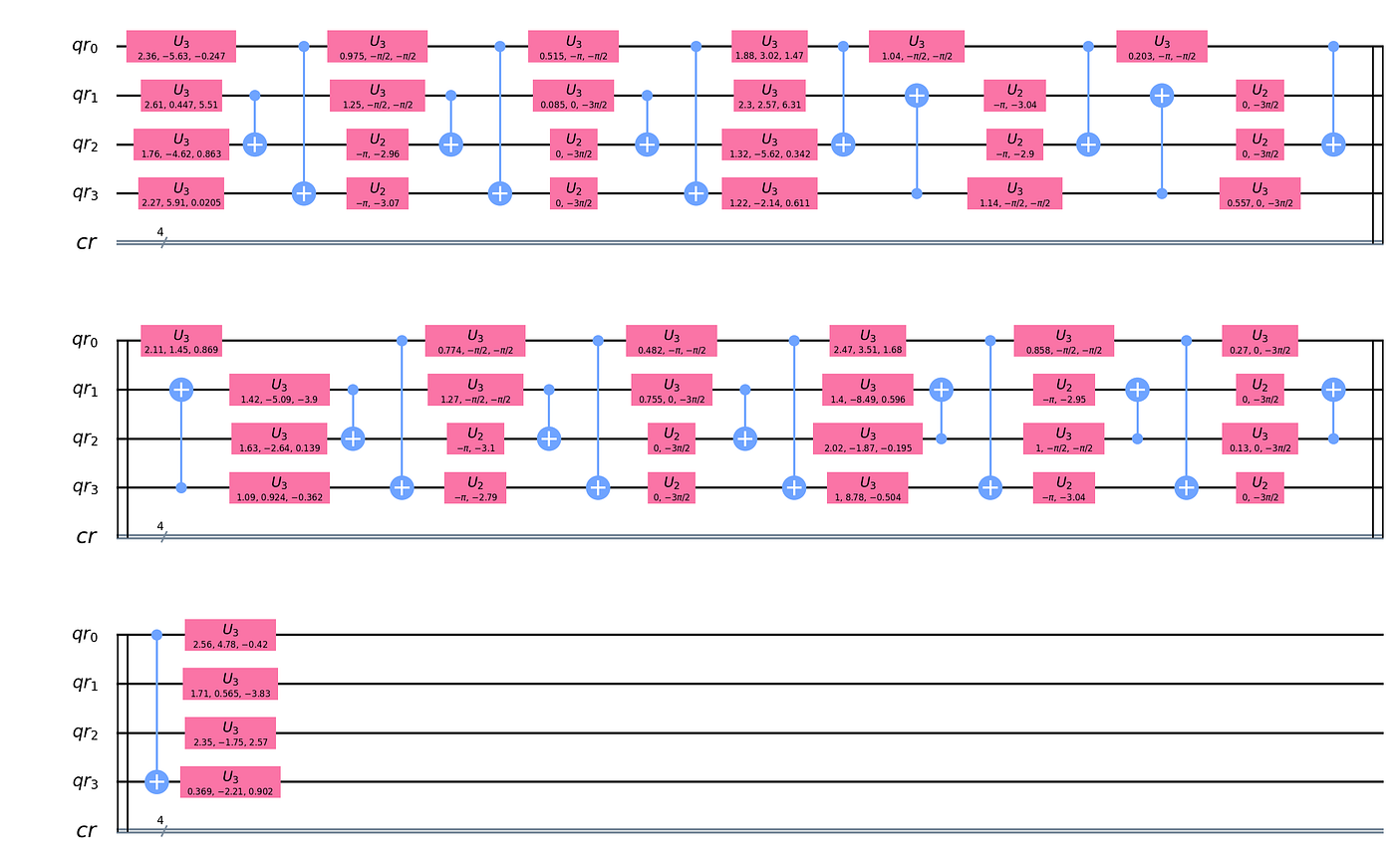What Is Quantum Volume, Anyway?A graph showing the number of IBM backends over time. Each bar is broken into the number of backends achieving each given Quantum Volume.

By Ryan F. Mandelbaum, Senior Technical Writer, IBM Quantum & Qiskit

Today, we announced that the IBM Quantum team achieved a Quantum Volume of 64 on one of its deployed systems. This is an exciting milestone, but the Quantum Volume measurement can be opaque even to folks who know how to program quantum computers. So, what is Quantum Volume and how do you calculate it?

Quantum Volume is a single number meant to encapsulate the performance of today’s quantum computers, like a classical computer’s transistor count. The IBM team has a committed roadmap to at least double the Quantum Volume of its devices annually, similar to how Moore’s law has driven the doubling of classical computer transistor count every two years. The IBM team is right on schedule with its Quantum Volume 64 announcement, representing the fourth time the team has doubled Quantum Volume in as many years.

First, in case you’re completely new here: A quantum computer is a kind of device that uses the mathematical rules obeyed by atoms, called quantum mechanics, to store and manipulate information. These computers run quantum circuits, which are like classical computers’ logic circuits but incorporate the quantum mechanical principles of superposition, entanglement, and interference in order to perform calculations off-limits to today’s most advanced supercomputers. Quantum computers represent this information as the quantum states of engineered systems called qubits, short for quantum bits, and manipulate these qubits by linking them into quantum circuits using quantum operations, called quantum gates.

The IBM team introduced the Quantum Volume metric specifically because a classical computer’s transistor count and a quantum computer’s quantum bit count aren’t the same. Qubits decohere, today forgetting their assigned quantum information in less than a millisecond. A quantum computer with a few, low-error, highly connected, and scalable qubits will probably bring us closer to the goal of a universal, fault-tolerant quantum computer — one capable of performing advanced quantum algorithms and powerful molecular simulations — than a device with lots of especially noisy, error-prone qubits would.

The Quantum Volume protocol tests how well a quantum computer can run a circuit consisting of random two-qubit gates acting in parallel on a subset of the device’s qubits. These circuits have a width, meaning how many qubits are involved, and a depth, meaning the number of discrete time steps during which the circuit can run gates before the qubits decohere. The protocol allows the quantum computer to rewrite or “transpile” the circuit into one that it can actually run based on its available gates and how its qubits are interconnected. The Quantum Volume protocol identifies the largest square-shaped circuit — one where the width and depth are equal — that can be run on a given quantum device.A diagram of the 27 qubit “Montreal” chip on which quantum volume was demonstrated, showing how its qubits are arranged. Each spot represents a qubit.

Quantum circuits can output multiple different strings of bits, including both a host of expected bit strings as well as unintended ones caused by qubit errors. The protocol determines whether a quantum computer running the given circuit is outputting the correct bit strings using the “heavy output generation problem,” which goes as follows: Each of the circuit’s correct output strings has an associated probability that you’ll measure it. You can find a median probability from that set of probabilities, and the “heavy outputs” of the circuit are all of those strings for which the probability of measuring them is greater than the median probability. The protocol first requires simulating the circuit on a classical computer to gather the heavy outputs. Then, you run the circuit lots of times on the device you want to benchmark, increment the circuit’s depth and width by one simultaneously, and run it again. The process stops at the highest depth and corresponding width for which the probability of measuring any of the heavy outputs on the device is greater than two-thirds with a confidence interval greater than 97.725% — basically, you have to successfully run the circuit enough times to ensure that your measurements aren’t a fluke.

Finally, raise 2 to the power of whatever the final depth was, and boom, that’s your Quantum Volume. The purpose of this last step is to give a sense of how rich a space of quantum states you have access to, and additionally, the time it takes to simulate this circuit with a classical computer approximately scales by 2 raised to the number of qubits.

For example: Let’s say that you have a 27-qubit quantum computer. You set up a random two-qubit circuit of depth two, run it lots of times, and the test succeeds — it outputs heavy strings with greater than two-thirds probability and with a confidence interval greater than 97.725%. You repeat with three qubits at depth three, four qubits at depth four, five qubits at depth five, and six qubits at depth six, and the test still works. If you move up to seven qubits with a circuit of depth seven and the test fails, then the Quantum Volume is 2⁶, or 64.

IBM successfully ran this exact test to achieve a Quantum Volume of 64 on its 27 qubit “Montreal” system — and the test didn’t even require building a new device. Instead, the team incorporated improvements to the Qiskit compiler, refined the calibration of the two-qubit gates, and issued upgrades to the noise handling and readout based on tweaks to the microwave pulses and gates before they’re applied in the circuit. You can read the paper detailing the achievement on the arXiv preprint server here.

You can run the Quantum Volume test on your own in Qiskit, as explained in the Learn Quantum Computation using Qiskit textbook, but to summarize: you begin by specifying which subset of qubits you’d like to run the test on, then generate the random circuits using these qubits. You use the qasm simulator to determine what the heavy outputs are. You run the circuit again on a quantum computer (or a simulator with a noise model added) and calculate the probability of measuring one of the heavy output strings and the confidence interval.A Quantum Volume circuit in Qiskit running on four qubits. The top four lines of the “music staff” represent a qubit. The bottom line represents the four classical bits that store the qubit value after it’s measured. Each “note” on the staff represents a quantum gate. This circuit has been transpiled to run on an IBM Quantum device.

It’s important to note that, while Quantum Volume is IBM’s preferred metric for benchmarking quantum computers, it doesn’t capture the entire complexity of a quantum device, such as how every qubit in the device performs. That’s the reason why the IBM team also publishes many additional measurements for each device and system. Additionally, we’ll eventually need to update the Quantum Volume metric once the quantum computers are too complex to find the heavy outputs classically. Such an update will likely rely on extended Clifford circuits — those involving only a subset of the gates which can be efficiently simulated with classical computers.

Hopefully this demonstrates two things: one, that Quantum Volume is a useful metric to compare today’s quantum computers, and two, that we’re still in early days of quantum computing, where it’s a challenge to get more than a handful of qubits to run long circuits. But that’s all part of the story. Moving from a Quantum Volume of 32 to 64 required viewing our devices as systems where we tweak everything that interacts with the qubits, from the compiler to the very shape of the microwave pulses that we send the qubits. Improvements to every part of these systems, from the chips themselves to the code used to run them, will play a crucial role as quantum computers mature.

Interested in trying to run Quantum Volume circuits on your own? Get started with Qiskit here.